Question

# Let ne Nj. Prove that n < 2(6(n)).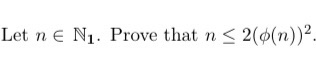Let ne Nj. Prove that n < 2(6(n)).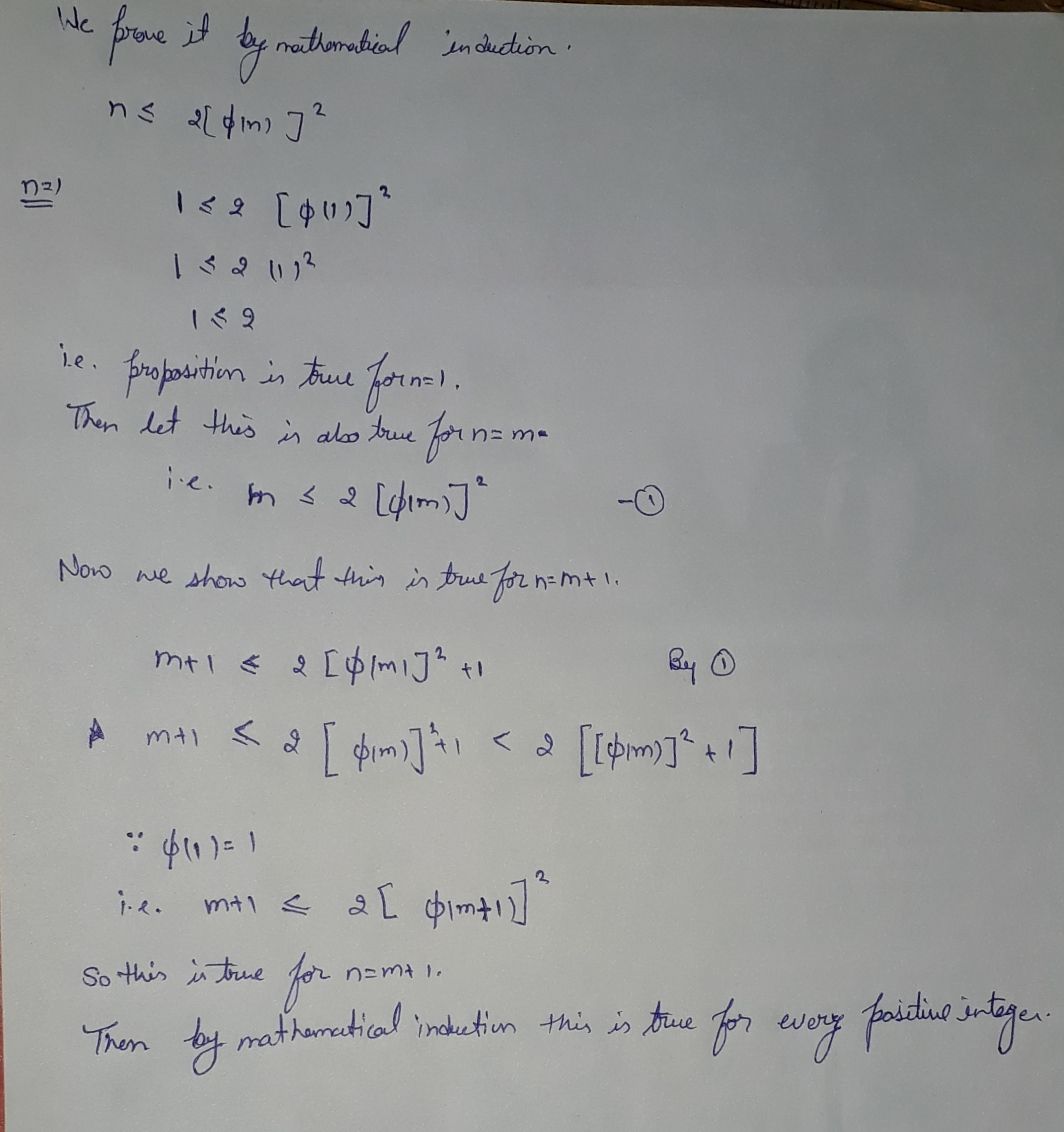#### Earn Coins

Coins can be redeemed for fabulous gifts.

Similar Homework Help Questions
• ### Let U ? Rmxn. Prove that if UTI-In, then n < m.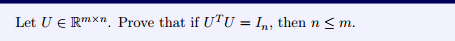Let U ? Rmxn. Prove that if UTI-In, then n < m.

• ### Let A be an mx n matrix and B be an n xp matrix. (a) Prove...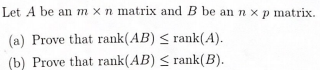Let A be an mx n matrix and B be an n xp matrix. (a) Prove that rank(AB) S rank(A). (b) Prove that rank(AB) < rank(B).

• ### let a,b > 0 . Prove that    DI < Val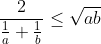let a,b > 0 . Prove that    DI < Val

• ### 2. (D5) Let n = o(a) and assume that a =bk. Prove that <a >=<b> if...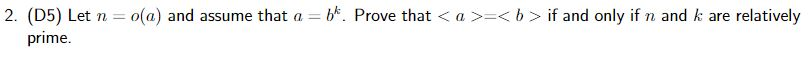2. (D5) Let n = o(a) and assume that a =bk. Prove that <a >=<b> if and only if n and k are relatively prime.

• ### 6. (20 pts) Prove that there is no homeomorphism of the closed interval (-1,1] of the...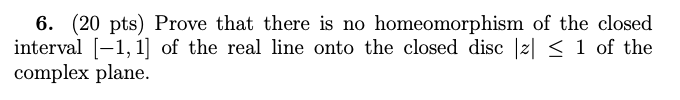6. (20 pts) Prove that there is no homeomorphism of the closed interval (-1,1] of the real line onto the closed disc < 1 of the complex plane.

• ### Exercise 1.25. This exercise relates to (1.13). Suppose that x > 1. For each ne N...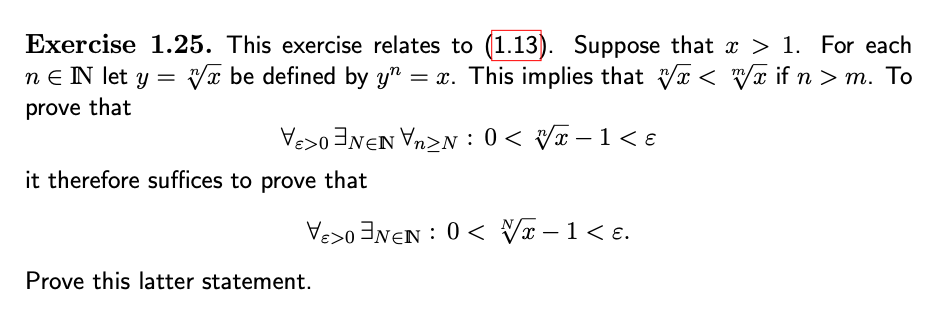Exercise 1.25. This exercise relates to (1.13). Suppose that x > 1. For each ne N let y= Vå be defined by yn = x. This implies that vx < mă if n > m. To prove that Veso 3NEN Vnən : 0< V2-1<e it therefore suffices to prove that Vesonen: 0< Vx-1<e. Prove this latter statement.

• ### IDY in < oo and lim - Yn < 0o. Prove that lim,+ 1. Let In...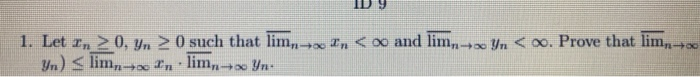IDY in < oo and lim - Yn < 0o. Prove that lim,+ 1. Let In > 0. Yn > 0 such that lim,- Yn) < lim,-- In lim,+ Yn: i tn < oo and lim yn < . Prove that lim. In 1. Let In 20, yn 0 such that lim Yn) < limn+In lim + Yr

• ### , then n lim Let Ά be a square matrix. Prove that if ρ(A)<1 Use the...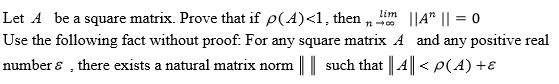, then n lim Let Ά be a square matrix. Prove that if ρ(A)<1 Use the following fact without proof. For any square matrix A and any positive real number ε , there exists a natural matrix norm I l such that l-4 ll < ρ (d) +ε IIA" 11-0

• ### 1 4.6.3. (Harder!) Let 0 < a < 1. Prove that for any n EN, (1...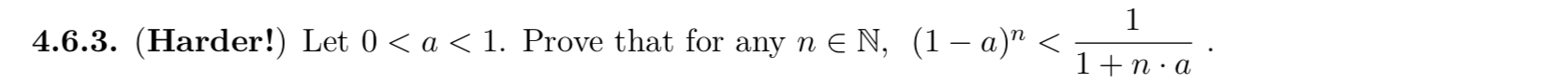1 4.6.3. (Harder!) Let 0 < a < 1. Prove that for any n EN, (1 – a)” < 1+n·a

• ### Theory 00 2. Prove that if Vlan] < 1 then an converges. n=1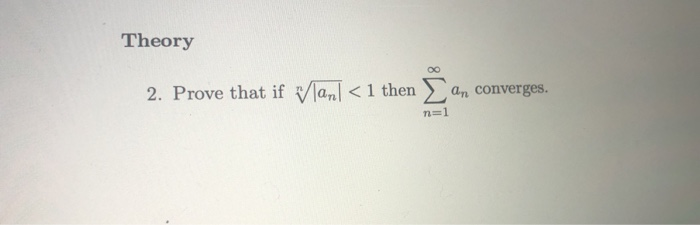Theory 00 2. Prove that if Vlan] < 1 then an converges. n=1